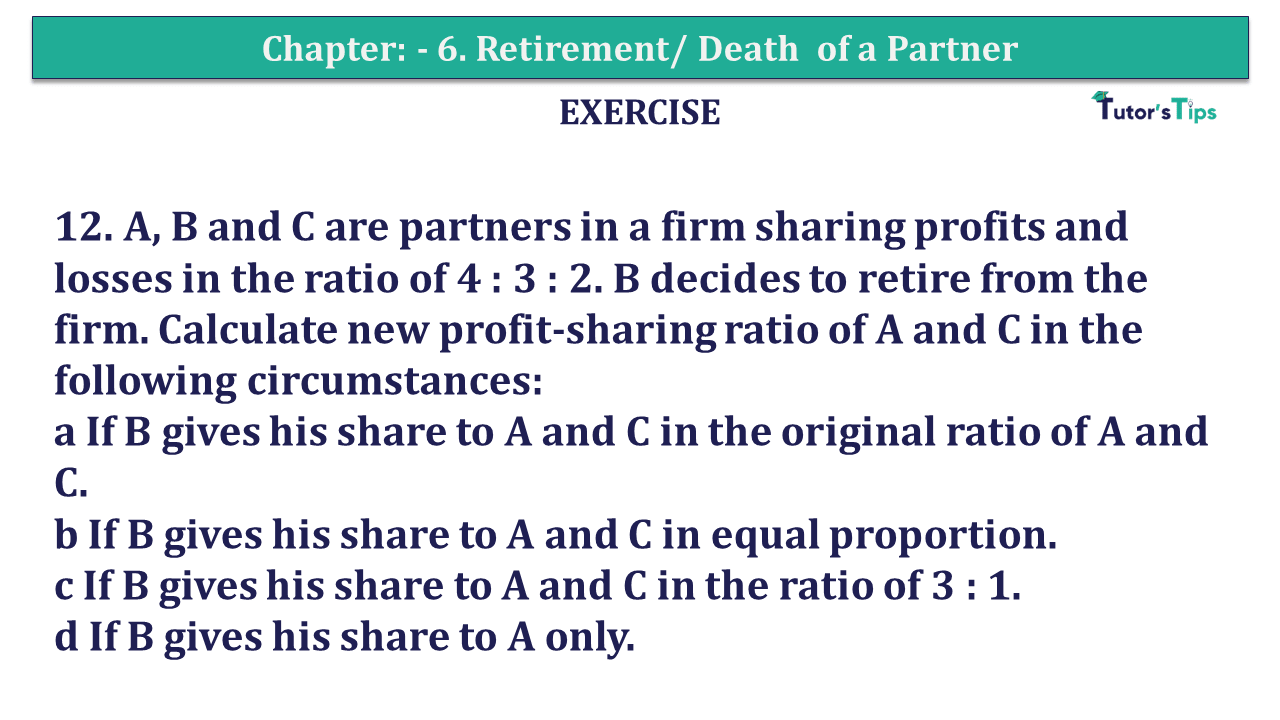# Question 12 Chapter 6 of +2-A – T.S. Grewal 12 Class Part – A Vol. 1Question No.12 Chapter No.6 - T.S. Grewal +2 Book 2019-Solution

Question 12 Chapter 6 of +2-A

12. A, B and C are partners in a firm sharing profits and losses in the ratio of 4 : 3 : 2. B decides to retire from the firm. Calculate new profit-sharing ratio of A and C in the following circumstances:
a If B gives his share to A and C in the original ratio of A and C.
b If B gives his share to A and C in equal proportion.
c If B gives his share to A and C in the ratio of 3 : 1.
d If B gives his share to A only.

## The solution of Question 12 Chapter 6 of +2-A: –

Old Ratio A, B and C = 4 : 3 : 2
B retires from the firm.

 His profit share = 3 9

Case a B gives his share to A and C in their original ratio.

Original Share A and C = 4 : 2

 Share taken by A = 3 X 4 9 6
 = 12 54

 Share taken by C = 3 X 2 9 6
 = 6 54

New Ratio = Old Ratio + Share acquired from B

 A’s New Share = 4 + 12 9 54
 = 24 + 12 54
 = 36 54

 C’s New Share = 2 + 6 9 54
 = 12 + 6 54
 = 18 54

∴ New Profit Ratio A and C = 36 : 18 or 2 : 1

Case b B gives his share to A and C in equal proportion

 Share taken by A = 3 X 1 9 2
 = 3 18

 Share taken by C = 3 X 1 9 2
 = 3 18

New Ratio = Old Ratio + Share acquired from B

 A’s New Share = 4 + 3 9 18

 = 8 + 3 18
 = 11 18

 C’s New Share = 2 + 3 9 18
 = 4 + 3 18
 = 7 18

∴ New Profit Ratio A and C = 11 : 7

Case c B gives his to A and C in the ratio 3 : 1.

 Share taken by A = 3 X 3 9 4

 = 9 36

 Share taken by C = 3 X 1 9 4
 = 3 36

New Ratio = Old Ratio + Share acquired from B

 A’s New Share = 4 + 9 9 36
 = 16 + 9 36

 = 25 36

 C’s New Share = 2 + 3 9 36
 = 8 + 3 36
 = 11 36

∴ New Profit Ratio A and C = 25 : 11

Case d B gives his share to A only

 A’s New Share = A’s Old Share + Share of B = 4 + 3 9 9
 = 7 9

 C’s Share = 2 9

∴ New Profit Ratio A and C = 7 : 2

T.S. Grewal’s Double Entry Book Keeping +2 (Vol. I: Accounting for Not-for-Profit Organizations and Partnership Firms)

• Chapter No. 1 – Financial Statement of Not-For-Profit Organisations
• Chapter No. 2 – Accounting for Partnership Firms – Fundamentals
• Chapter No. 3 – Goodwill: Nature and Valuation
• Chapter No. 4 – Change in Profit-Sharing Ratio Among the Existing Partners
• Chapter No. 5 – Admission of a Partner
• Chapter No. 6 – Retirement/Death of a Partner
• Chapter No. 7 – Dissolution of a Partnership Firm

### T.S. Grewal’s Double Entry Book Keeping (Vol. II: Accounting for Companies)

• Chapter No. 1 – Financial Statements of a Company
• Chapter No. 2 – Financial Statement Analysis
• Chapter No. 3 – Tools of Financial Statement Analysis – Comparative Statements and Common- Size Statements
• Chapter No. 4 – Accounting Ratios
• Chapter No. 5 – Cash Flow Statement